## 5.2Gauss-Seidel method

Finite volume numerics generally uses iterative methods to solve each matrix equation. These methods calculate approximate solutions for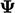, which become more accurate with successive iterative solutions.

Iterative methods are preferred because they are more eﬃcient than direct methods, which solve a matrix equation exactly. Gaussian elimination, which is the numerical basis for direct solution methods, has a computational cost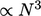. This is prohibitive for many sizes of mesh in ﬁnite volume CFD.

Gauss-Seidel1 is a simple, iterative method which is generally eﬀective for solving transport equations such as the example in Sec. 5.1 . The method is illustrated by a sample equation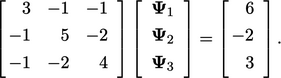(5.2)
The equations can be rewritten by: a) multiplying the oﬀ-diagonal coeﬃcients by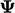; b) subtracting the result from the right hand side (r.h.s.); c) and, dividing by the diagonal coeﬃcient. i.e.: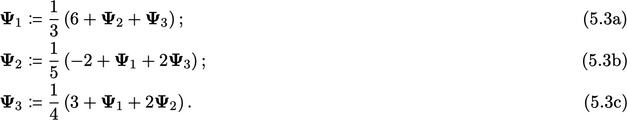Starting with,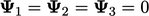, new values ofare calculated by Eq. (5.3a ), Eq. (5.3b) and Eq. (5.3c ) in sequence, where the notation “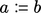” denotes “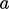is assigned the value of.

The ﬁrst solution of Eq. (5.3a) is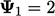. The updated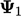is substituted in Eq. (5.3b), whose solution is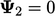. Both updated values are substituted in Eq. (5.3c ) to give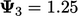.

The process is then repeated and through successive sweeps over the equations the solution converges as shown below.

 Variable Start Sweep 1 …2 …3 …4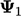0.0000 2.0000 2.4167 2.7431 2.8821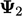0.0000 0.0000 0.5833 0.8069 0.9121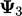0.0000 1.2500 1.6458 1.8392 1.9266
 Variable Sweep 5 …6 …7 …8 …92.9462 2.9755 2.9888 2.9949 2.9977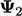0.9599 0.9817 0.9916 0.9962 0.99831.9665 1.9847 1.9930 1.9968 1.9985

The error is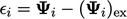, i.e. the diﬀerence between the approximate and exact values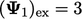,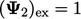and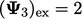. After 9 sweeps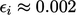for all variables, i.e. within  0.2% of the exact solution.

In summary, the Gauss-Seidel method is the following sequence of calculations for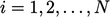, repeated until convergence: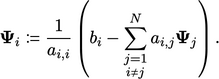(5.4)
The Gauss-Seidel method, applied to sparse matrices, has a computational cost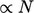, making it practical for simulations with large meshes that often occur in ﬁnite volume CFD.

Convergence of the method, and convergence measures for iterative methods in general, are discussed in the following sections.

1Carl Friedrich Gauss, letter to Christian Gerling, 1823.

Notes on CFD: General Principles - 5.2 Gauss-Seidel method